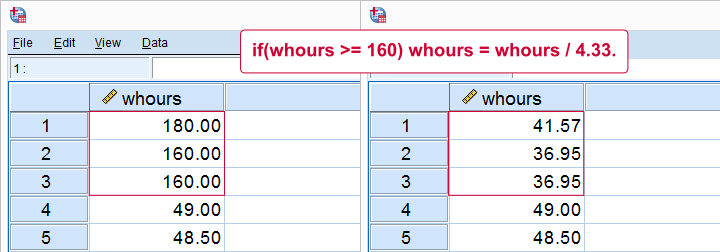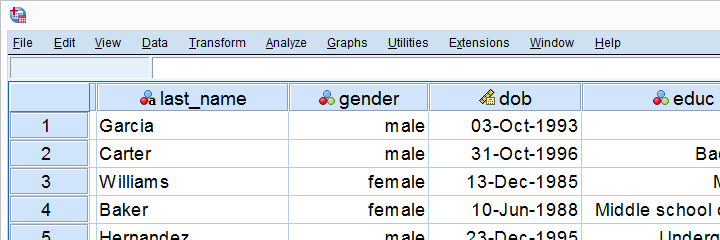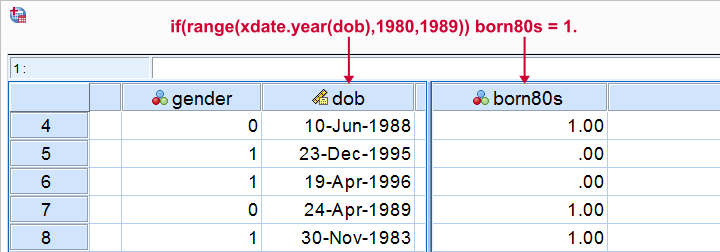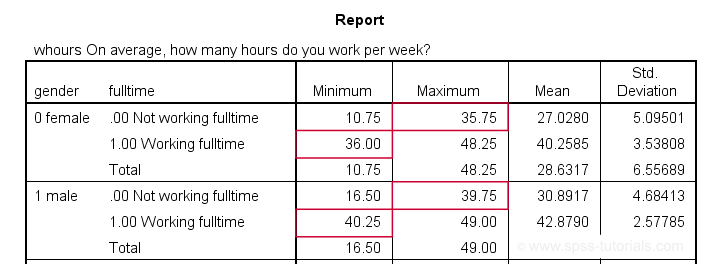SPSS TUTORIALS BASICS ANOVA REGRESSION FACTOR CORRELATION

# SPSS IF – A Quick Tutorial

In SPSS, IF computes a new or existing variable
for a selection of cases.
For analyzing a selection of cases, use FILTER or SELECT IF instead.## Data File Used for Examples

All examples use bank.sav, a short survey of bank employees. Part of the data are shown below. For getting the most out of this tutorial, we recommend you download the file and try the examples for yourself.## Example 1 - Flag Cases Based on Date Function

Let's flag all respondents born during the 80’s. The syntax below first computes our flag variable -born80s- as a column of zeroes. We then set it to one if the year -extracted from the date of birth- is in the RANGE 1980 through 1989.

*Create new variable holding only zeroes.
compute born80s = 0.

*Set value to 1 if respondent born between 1980 and 1989.
if(range(xdate.year(dob),1980,1989)) born80s = 1.
execute.

add value labels born80s 0 'Not born during 80s' 1 'Born during 80s'.

## Result## Example 2 - Replace Range of Values by Function

Next, if we'd run a histogram on weekly working hours -whours- we'd see values of 160 hours and over. However, weeks only hold (24 * 7 =) 168 hours. Even Kim Jong Un wouldn't claim he works 160 hours per week!
We assume these respondents filled out their monthly -rather than weekly- working hours. On average, months hold (52 / 12 =) 4.33 weeks. So we'll divide weekly hours by 4.33 but only for cases scoring 160 or over.

*Sort cases descendingly on weekly hours.
sort cases by whours (d).

*Divide 160 or more hours by 4.33 (average weeks per month).
if(whours >= 160) whours = whours / 4.33.
execute.

## Result## Note

We could have done this correction with RECODE as well: RECODE whours (160 = 36.95)(180 = 41.57). Note, however, that RECODE becomes tedious insofar as we must correct more distinct values. It works reasonably for this variable but IF works great for all variables.

## Example 3 - Compute Variable Differently Based on Gender

We'll now flag cases who work fulltime. However, “fulltime” means 40 hours for male employees and 36 hours for female employees. So we need to use different formulas based on gender. The IF command below does just that.

*Compute fulltime holding only zeroes.
compute fulltime = 0.

*Set fulltime to 1 if whours >= 36 for females or whours >= 40 for males.
if(gender = 0 & whours >= 36) fulltime = 1.
if(gender = 1 & whours >= 40) fulltime = 1.

add value labels fulltime 0 'Not working fulltime' 1 'Working fulltime'.

*Quick check.
means whours by gender by fulltime
/cells min max mean stddev.

## Result

Our syntax ends with a MEANS table showing minima, maxima, means and standard deviations per gender per group. This table -shown below- is a nice way to check the results.The maximum for females not working fulltime is below 36. The minimum for females working fulltime is 36. And so on.

## SPSS IF Versus DO IF

Some SPSS users may be familiar with DO IF. The main differences between DO IF and IF are that

• IF is a single line command while DO IF requires at least 3 lines: DO IF, some transformation(s) and END IF.
• IF is a conditional COMPUTE command whereas DO IF can affect other transformations -such as RECODE or COUNT- as well.
• If cases meet more than 1 condition, the first condition prevails when using DO IF - ELSE IF. If you use multiple IF commands instead, the last condition met by each case takes effect. The syntax below sketches this idea.

## DO IF - ELSE IF Versus Multiple IF Commands

*DO IF: respondents meeting both conditions get result_1.
do if(condition_1).
result_1.
else if(condition_2). /*excludes cases meeting condition_1.
result_2.
end if.

*IF: respondents meeting both conditions get result_2.
if(condition_1) result_1.
if(condition_2) result_2. /*includes cases meeting condition_1.

## SPSS IF Versus RECODE

In many cases, RECODE is an easier alternative for IF. However, RECODE has more limitations too.
First off, RECODE only replaces (ranges of) constants -such as 0, 99 or system missing values- by other constants. So something like recode overall (sysmis = q1). is not possible -q1 is a variable, not a constant- but if(sysmis(overall)) overall = q1. works fine. You can't RECODE a function -mean, sum or whatever- into anything nor recode anything into a function. You'll need IF for doing so.

Second, RECODE can only set values based on a single variable. This is the reason why you can't recode 2 variables into one but you can use an IF condition involving multiple variables: if(gender = 0 & whours >= 36) fulltime = 1. is perfectly possible.

You can get around this limitation by combining RECODE with DO IF, however. Like so, our last example shows a different route to flag fulltime working males and females using different criteria.

## Example 4 - Compute Variable Differently Based on Gender II

*Recode whours into fulltime for everyone.
recode whours (40 thru hi = 1)(else = 0) into fulltime2.

*Apply different recode for female respondents.
do if(gender = 0).
recode whours (36 thru hi = 1)(else = 0) into fulltime2.
end if.

add value labels fulltime2 0 'Not working fulltime' 1 'Working fulltime'.

*Quick check.
means whours by gender by fulltime2
/cells min max mean stddev.

## Final Notes

This tutorial presented a brief discussion of the IF command with a couple of examples. I hope you found them helpful. If I missed anything essential, please throw me a comment below.

# Tell us what you think!

*Required field. Your comment will show up after approval from a moderator.

# THIS TUTORIAL HAS 53 COMMENTS:

•### By godfrey matumu on November 20th, 2014

However, i have one thing that troubles me.
I have spss db, it has a variable date of death with the format of dd.mm.yyyy.
I want to change this into yes or no.
how do I do it with the if statement?

•### By Ruben Geert van den Berg on November 20th, 2014

There's a faster way than `IF` for doing so and it's discussed here. Regarding the date comparison, use the `DATE.DMY` function. I wrote a tiny example of this and put it here.

HTH,

Ruben

•### By shimaa ahmed on March 23rd, 2015

i wanna to know how can i do this statement on spss syntax windows (if the answer in Q5 'No' , transfer to Q7)
and (Age ranges between 18 and 60)

•### By Ruben Geert van den Berg on March 23rd, 2015

See RANGE.

•### By Van on June 22nd, 2015

Dear Ruben
Thank you for the useful tutorial.
I've been wondering if you actually know some trick in SPSS to deal the following issue:
I have a task consisting of 30 trials, this means that each subject provides 30 answers. Now I need to calculate the mean of the reaction time but only for correct trials. Also, the order of these trials are randomized for all subjects and the randomization order is unfortunately not recorded by the program I use for collecting data.
At the moment I don't know how to do this the fastest way in SPSS.
What I am doing now is first I pick out the correct answers by telling SPSS to recode the original RT variables into new variables (called RT_correctanswer, for example) if each of them satisfies the condition that the corresponding Trial_correct variable is correct (1 = correct, 0 = incorrect). If the condition is not satisfied then the new variable just has a missing value. This means that I will have 30 new RT_correctanswer variables. Then I ask SPSS to take the mean of these RT_correctanswer variables. This method works fine for one dataset and for one thing to filter out (correct vs. incorrect). Now I have a few data sets with different number of trials and there are also a bunch of other things I have to filter out which entails a lot more syntax composing than I can manage without making some serious mistakes here and there. I wonder if you know anything better?
The problem lies in the condition, I don't know how to tell SPSS to do the following:
If Trial1_correct = 1 and Trial2_correct = 1 and ...
Then compute Mean_RT = MEAN(Trial1_RT, Trial2_RT, ...).
The thing is SPSS will not compose any mean. Because no one has perfect 30 correct answers. What I would like it to do is to calculate the mean RT but only for trials where the associated Correct variable is 1 and ignore others. I tried to change "and" to "or" but then only get error messages.